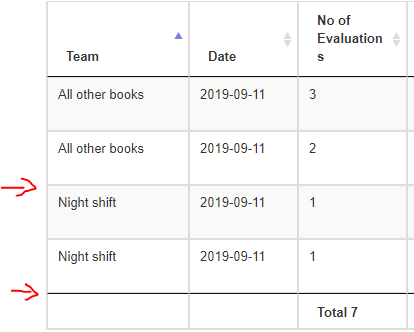# KoolReport's Forum

Official Support Area, Q&As, Discussions, Suggestions and Bug reports.

Frederic Begue opened this topic on on Sep 11, 2019 - 14 comments

Frederic Begue commented on Sep 11, 2019

Hi,

Is there a way to add subtotal in Tables/DataTables?KoolReport commented on Sep 11, 2019

You use the "grouping" feature of Table and set the "bottom" property to show subtotal at bottom of rows group. Here is the example.

paulo commented on Jun 18

Hi, How Can we have the same feature with sum/totals at the bottom using DataTables ? thanks

David Winterburn commented on Jun 19

Hi Paulo,

Did you mean sum total in footer of DataTables or sum total with row group in DataTables?

paulo commented on Jun 19

Hi David, subtotals on each category / grouped by . Same way you have here: https://www.koolreport.com/examples/reports/koolphp_table/row_group/

but using the Datagrid. i.e. Total of year 2004 \$4,313,328

thanks

David Winterburn commented on Jun 22

Hi Paulo,

For DataTables' rowgroup please try this client-side solution first:

https://datatables.net/extensions/rowgroup/examples/initialisation/startAndEndRender.html

The code for creating DataTables widget is like:

``````<?php
DataTables::create(arrary(
...
"options" => array(
"order" => [[2, 'asc']],
"dataSrc" => 2,
"endRender" => "function ( rows, group ) {
var avg = rows
.data()
.pluck(5)
.reduce( function (a, b) {
return a + b.replace(/[^\d]/g, '')*1;
}, 0) / rows.count();

return 'Average salary in '+group+': '+
\$.fn.dataTable.render.number(',', '.', 0, '\$').display( avg );
}"
),
));
``````

We will add rowGroup server-side option in the future for simpler setup. Thanks!

paulo commented on Jun 23

thank you Server-side would be great This totaling worked, but the totals are showing without formatting and without decimal places I have row 1: 261056 row 2: \$48.06 The total of this grouping : 261056 Plus it is not right-aligning .... I've this tha works for all Datatables without grouping:

``````        "cssClass" => array(
"table" => "table-bordered table-striped table-hover cell-border compact",
'tf' => "text-right",
"td"=>function(\$row,\$colName) {
{
return "text-right";
}
},
)
``````

thank you

David Winterburn commented on Jun 24

Hi Paulo,

To format a string/number to number format with comma please try this js function:

``````function numberWithCommas(x) {
return x.toString().replace(/\B(?<!\.\d*)(?=(\d{3})+(?!\d))/g, ",");
}
``````

Another option is to use js native function:

``````let formattedNum = num.toLocaleString();
``````

I've tested these to format numbers on client side and they work for my case. Thanks!

paulo commented on Jun 24

I tried replacing the line +avg.toFixed(0)+ with both examples and it won't show decimals, etc

David, this seems to be a bit more complicated than most of the koolreports, I am wondering if eventually the plan is to move this into a more simple approach like Tables grouping....

thanks

"endRender" => "function ( rows, group ) {

``````                                    var avg = rows
.data()
.pluck(3)
.reduce( function (a, b) {
return a + b.replace(/[^\d]/g, '')*1;
}, 0);

return \$('<tr/>')
.append( '<td colspan=\"3\">Total for '+group+'</td>' )
.append( '<td>'+avg.toFixed(0)+ '</td>' )
.append( '<td/>' );
``````
``````                          }"
``````
David Winterburn commented on Jun 25

Of course avg.toFixed(0) will remove the decimal part. If you want to show, says, 2 decimals number please try avg.toFixed(2). Thanks!

paulo commented on Jun 25

David, I tried both ways. I have two rows \$2,562.50 + \$48.06 with avg.toFixed(0) , total is 261056 with avg.toFixed(2) total is 261056.00 Here is my whole endRender function:

"endRender" => "function ( rows, group ) {

``````                                    var avg = rows
.data()
.pluck(3)
.reduce( function (a, b) {
return a + b.replace(/[^\d]/g, '')*1;
}, 0);

return \$('<tr/>')
.append( '<td colspan=\"3\">Total for '+group+'</td>' )
.append( '<td>'+avg.toFixed(0)+ '</td>' )
.append( '<td/>' );
``````
``````                          }"
``````

Is there anyway I can do this through the PHP side ? Sorry for insisting, I am hopping to stick to php as this is a PHP Reporting Framework.

Or do you have any other suggestion ? is there anything I can replace above that would fix the issue? If I do: .reduce( function (a, b) {

``````                                            return a + b.replace(/\B(?<!\.\d*)(?=(\d{3})+(?!\d))/g, ",")*1;
}, 0);
``````

I get NaN

thank you

David Winterburn commented on Jun 26

``````b.replace(/[^\d]/g, '')*1
``````

with:

``````b.replace(/[^\d\.]/g, '')*1
``````

We will add server-side row group feature for DataTables in the future. Thanks!

paulo commented on Jun 26

Thank you very much David! Now, it shows " 2610.56" avg.toFixed(2) ! which is great. Is there a function to format properly with comma ? I added \$ as string. \$2610.56 .append( '<td class="text-right">\$'+avg.toFixed(2)+ '</td>' )

thank you

David Winterburn commented on Jun 29

``````function numberWithCommas(x) {
return x.toString().replace(/\B(?<!\.\d*)(?=(\d{3})+(?!\d))/g, ",");
}
``````

or:

``````let formattedNum = num.toLocaleString();
``````

Thanks!

paulo commented on Jun 30

perfect! it worked and formatted properly. Thank you very much for your help !